Select Page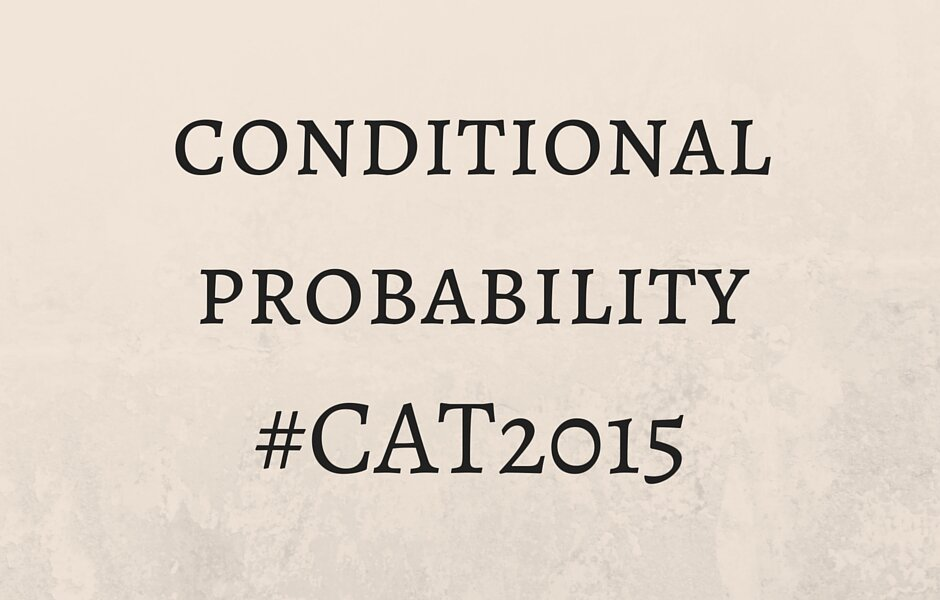Probability brings along with it a certain amount of dreadfulness. Even the most confident of aspirants will probably (what else) think twice before marking an option for a permutations and combinations or a probability question. While thinking about probability questions as if they were a story generally helps crack even the most difficult of questions, conditional probability is a sparsely explored concept which might appear as a moderate-to-difficult level question.

What is conditional probability?

As the name suggests, it is nothing but probability of the occurrence of a favourable event under another preceding condition. So essentially, the definition of probability remains the same but you have to consider that the chances of the occurrence of the event will be a subset of the total probability.

Probability of intersection of independent events

Let’s assume that there is a probability of 1/10 of a person being a hypnotist. At the same time, there is another stat that tells that there is a probability of 1/5 of a person having blue eyes. As these are independent events (in a non Yo Yo Honey Singh world, of course) the probability of a person being a hypnotist AND having blue eyes is simply the multiplication of the two probabilities i.e. 1/10*1/5 = 1/50.

So, for independent events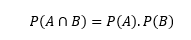Question types

Simply put, a conditional probability question would mention a story in reverse. It will give you an outcome and then ask you what would be the probability that the event would have had a particular predecessor. As there is no other question type associated with conditional probability, many would feel that it is too much of an effort to put into a single question which hasn’t really been frequenting the CAT papers. However, the questions are easy and as is true with any probability question there isn’t much calculation involved.

A question from this area would go like:

A teacher gave her class two tests. 30% of the class passed both tests and 50% of the class passed the first test. What percent of those who passed the first test also passed the second test?

Now, there is a formula based approach to this and a simpler approach based on pure understanding. For the formula-philes, here we go: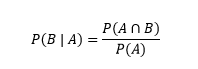For those who prefer a logical approach, you can proceed as follows: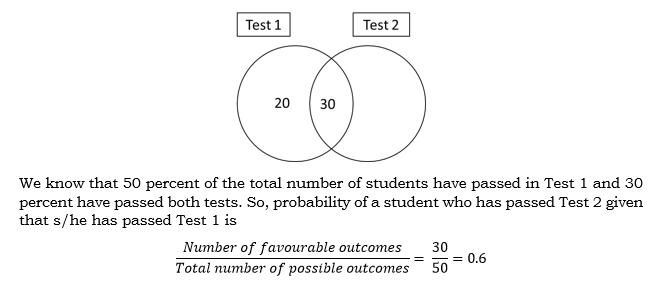Sounds simpler now?

Bayes’ theorem

It is simply an extension of the definition of conditional probability.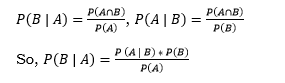The complicated type

The above example was easy and is something which could be expected in an easy-moderate paper. However, there is another type which could come as a scorcher and would require a lot of thought while arriving at the answer. While it is not recommended to solve this question type right at the start, you can come to it if you have a round 2.

A bag contains 6 balls of one or more colours. A ball is picked and is found to be red. What’s the probability that the bad initially had exactly 6 red balls?

1. 2/7
2. 1/5
3. 3/5
4. 4/5

In this type, you have to understand that the probability of each preceding event is not equally distributed. In other words, there could have been 6 different predecessors to the event:

1. 6 red 0 different
2. 5 red 1 different
3. 4 red 2 different
4. 3 red 3 different
5. 2 red 4 different
6. 1 red 5 different

The probability of drawing a red ball in each of these cases is:

1. 6 red 0 different = 6c1/6c1 = 1
2. 5 red 1 different = 5c1/6c1 = 5/6
3. 4 red 2 different = 4c1/6c1 = 4/6
4. 3 red 3 different = 3c1/6c1 = 3/6
5. 2 red 4 different = 2c1/6c1 = 2/6
6. 1 red 5 different = 1c1/6c1 = 1/6

Now, all the individual probabilities form the sample set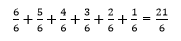So, probability of an individual event is given by:

1. 6 red 0 different = (1)/(21/6) = 6/21
2. 5 red 1 different = (5/6)/(21/6) = 5/21
3. 4 red 2 different = (4/6)/(21/6) = 4/21
4. 3 red 3 different = (3/6)/(21/6) = 3/21
5. 2 red 4 different = (2/6)/(21/6) = 2/21
6. 1 red 5 different = (1/6)/(21/6) = 1/21

As we are required to find the probability of the first case only, we get 2/7 as the answer.

Another similar question which goes another step ahead:

There is a mathemagician who has 4 cards hidden inside his cuff. The cards could be either aces or kings. Two cards accidentally fall out of his cuff during an act and they are found to be kings. He quickly manages the situation and places the cards back in their original position. But, as luck would have it, a card falls out again. What is the probability that this card is an ace?

(a) ½            (b) ¼            (c) 1/8         (d) 1/16

• 4 kings 0 aces = 1
• 3 kings 1 ace = 3c2/4c2 = 1/2
• 2 kings 2 aces = 2c2/4c2 = 1/6

That is the sample set (total = 10/6) and so, probability of individual events is:

• 4 kings 0 aces = (1)/(10/6) = 6/10
• 3 kings 1 ace = (1/2)/(10/6) = 3/10
• 2 kings 2 aces = (1/6)/(10/6) = 1/10

Probability of getting an ace is nothing but the product of the probability of the occurrence of a predecessor and the probability of the expected outcome.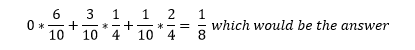You can practice a few questions from this topic

1. In Mumbai, 48% of all working professionals own a TV set and 39% of all working professionals own both a laptop and a TV set. What is the probability that a working professional owns a laptop given that the working professional owns a TV set?
2. A group of 10 people headed for a party, where they must take butter, jam, or both. If 3 people took butter, and 10 people took jam, then what percentage of the group took both?
3. Silent melody sells only two special models of flutes to their customers. Model A is purchased by 45% of their customers. Model B is purchased by 60% of their customers. What is the probability that a customer selected at random has purchased both Models A and B?
4. Ross and Rachel are a married couple with two children. One of the children is a boy. What is the probability that the other child is also a boy assuming that there is equal chance of an individual being born as a boy or a girl?
5. Arun and Barun are playing a game of dice. Each rolls one die and the one with higher number wins. If the numbers are the same, they roll again. If Arun just won, what is the probability that he had rolled a 5?

Edit: The answers to the questions are as follows:

1. 39/48

2. 30%

3. 5%

4. 1/3

5. 4/15

And finally, the classical Monty Hall problem:

Suppose you’re on a game show, and you’re given the choice of three doors: Behind one door is a car; behind the others, goats. You pick a door, say No. 1, and the host, who knows what’s behind the doors, opens another door, say No. 3, which has a goat. He then says to you, “Do you want to pick door No. 2?” Is it to your advantage to switch your choice?

Hope I have managed to clear a few doubts regarding conditional probability. Let us know if you have any other approach to these question types.

All the best!

error: Content is protected !!